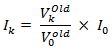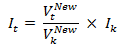6464.0 - Residential Property Price Indexes: Concepts, Sources and Methods, 2018
ARCHIVED ISSUE Released at 11:30 AM (CANBERRA TIME) 19/03/2019
Page tools:Print All

INTRODUCTION

9.1 It is important that ABS price indexes are reviewed regularly to ensure the indexes are relevant and accurately represent price movements in the market.

9.2 This section describes the reference periods used in the indexes and the chain linking process.

REFERENCE PERIODS

9.3 The following reference periods are discussed in this section:

• Weight reference period is the period covered by the expenditure statistics used to calculate the weights
• Price reference period is the period for which prices are used as denominators in the index calculation
• Index reference period is the period for which the index is set to 100.0.

RE-REFERENCING

9.4 The Australian Bureau of Statistics (ABS) changes the index reference period (a process known as re-referencing) of the indexes from time to time, but not frequently. This is because frequently changing the index reference period is inconvenient for users, particularly those who use the indexes for contract escalation.

9.5 The conversion of index numbers from a previous index reference period to a new index reference period involves rescaling the index numbers. This is achieved by applying conversion factors to the previous period index reference period series. Conversion factors are calculated by the ratio of the index numbers on the previous index reference period (2003-04 = 100.0) to the index numbers on the new index reference period (2011-12 = 100.0). Index numbers for financial years are calculated as the simple (arithmetic) averages of the four quarterly index numbers for that financial year.

 TABLE 5 RPPI, weighted average of eight capital cities, index numbers Period Index reference period 2003-04=100 (old) Index reference period 2011-12=100.0 (new) 2010-11March quarter 2011 145.5 102.7June quarter 2011 144.4 102.0 2011-12September quarter 2011 141.9 100.2December quarter 2011 140.8 99.4March quarter 2012 141.5 100.0June quarter 2012 142.1 100.4Financial year 2011-12 (a)141.6 (b)100.0 (a) Index number for financial year 2011-12 (index reference period 2003-04 =100.0) = (141.9+140.8+141.5+142.1)/4 =141.6 (b) Index number for financial year 2011-12 (index reference period 2011-12 =100.0) = (100.2+99.4+100.0+100.4)/4 = 100.0

9.6 A conversion factor is calculated as follows:
Rounded conversion factor = 100.0/141.6 = 0.7062
Index number for the September quarter 2011 (index reference period 2003-04 = 100.0) = 141.9
Index number for the September quarter 2011 (index reference period 2011-12 = 100.0) = 141.9 x 0.7062 = 100.2

9.7 Similarly, to convert index numbers on the new index reference period back to the old index reference period will also require rescaling of index numbers. The conversion factors to achieve this are obtained by taking the inverse of the previously described conversion factor.

9.8 Using the example above, a conversion factor is calculated as follows:
Rounded conversion factor = 141.6/100.0 = 1.416
Index number for the September quarter 2011 (index reference period 2011-12 = 100.0) = 100.2
Index number for the September quarter 2011 (index reference period 2003-04 = 100.0) = 100.2 x 1.416 = 141.9

Effect of rounding

9.9 Published percentage changes to index numbers are calculated from the rounded index numbers. A consequence of re-referencing price indexes can be that period-to-period percentage changes may differ to those previously published due to rounding of the re-referenced values. These differences do not constitute a revision.

INCORPORATING A REVIEW INTO AN INDEX

9.10 To incorporate new weights and updated stratification into an existing price index three steps must be undertaken: a link period must be chosen, value data must be price updated to the link period, and the newly weighted index chained onto the existing price index.

9.11 The link period for the indexes is the quarter in which the index is calculated on both the old weights and structure and the new weights and structure. The choice of link period is determined by a number of factors, but the aim is to avoid any periods where economic behaviour may be influenced by an abnormal event, such as regulatory changes.

9.12 The link period for the latest review is the September quarter 2018. This means that indexes from the December quarter 2018 onwards were calculated from the index for September quarter 2018, but with updated stratification and weighting patterns. This period also becomes the new price reference period for the indexes.

Price updating value data

9.13 When linking price indexes to implement a review or re-weight, the link period is usually different from the period for which the new value weights have been calculated. It is therefore necessary to price update the values from the weight reference period to the price levels of the link period.

9.14 The method for calculating price updated link period values for each stratum in the property price indexes differs to that of other ABS price indexes because actual quantities for the weight reference period are available. In other indexes, a measure of price change between the link period and the weight reference period is derived, and this is multiplied by the value or expenditure weights from the weight reference period. The updated value aggregates are aggregated to determine the upper level value aggregates.

9.15 In the HPI and ADPI the updated value of the dwelling stock is determined by multiplying the dwelling counts obtained from Census data by a measure of average price for the link period (the 'mean-adjusted median' (footnote 17)) for each strata. Although this method is different to that employed by other indexes described above, it delivers a mathematically equivalent result.

9.16 Chain linking can best be illustrated by means of an example. In this example consider a price index at period k, with the index constructed from weights introduced in the price reference period 0. Using the terminology from Calculation in Practice, the price index at period k is expressed as9.17 If period k is the link period, any future period price indexes will make comparisons back to the link period, and be scaled by the link period index Ik. Any such comparisons will use the same price index Ik but use a value aggregate calculated on the new weighting basis. If t is some period after k, a price index measuring the average price change from period 0 to period t is given by9.18 In the below example, in Periods 0 and 1, City A has been made up of three strata. The value aggregates are used to construct the index by taking the price relative between the value aggregate in the current period and the price reference period (period 0) and multiplying it by the index number in the price reference period. In Period 2, City A undergoes a re-stratification, and it is determined that four strata will be used for the index structure in the future.

9.19 In Period 2, value aggregates are calculated on both the old (Series 1) and new (Series 2) index structures. Thus Period 2 is the link period (or period k in the formula above) and will be the new price reference period.

9.20 To maintain a long time series, chain linking is used. In this case, the price index for the price reference period for Series 2 for City A is set as the Period 2 price index for Series 1. In Period 3, updated value aggregates for Series 2 are then used to calculate the index. Again, this is done by taking the price relative of the value aggregates and multiplying it by the index number in the price reference period, but now the price reference period is the link period (period 2).

9.21 It can be seen that the movements in the value aggregates are always reflected by the movement in the indexes. Thus, chain linking allows for a process of updating and maintaining the relevance of the indexes (in this case by re-stratification) whilst still allowing for a long time series.

 TABLE 6, Chain Linking ExamplePeriod 0 Period 1 Period 2 (Link Period) Period 3 Period 4 Period 5VALUE AGGREGATES SERIES 1Stratum 1 500.0 550.0 575.0 na na na Stratum 2 600.0 610.0 610.0 na na na Stratum 3 650.0 640.0 630.0 na na na City A 1 750.0 1 800.0 1 815.0 na na na % change na 2.9 0.8 na na naINDEX SERIES 1Stratum 1 100.0 110.0 115.0 na na na Stratum 2 100.0 101.7 101.7 na na na Stratum 3 100.0 98.5 96.9 na na na City A 100.0 102.9 103.7 na na na % change na 2.9 0.8 na na naVALUE AGGREGATES SERIES 2Stratum W na na 400.0 410.0 420.0 430.0 Stratum X na na 500.0 490.0 500.0 520.0 Stratum Y na na 600.0 620.0 625.0 630.0 Stratum Z na na 700.0 700.0 705.0 710.0 City A na na 2 200.0 2 220.0 2 250.0 2 290.0 % change na na na 0.9 1.4 1.8INDEX SERIES 2Stratum W na na 100.0 102.5 105.0 107.5 Stratum X na na 100.0 98.0 100.0 104.0 Stratum Y na na 100.0 103.3 104.2 105.0 Stratum Z na na 100.0 100.0 100.7 101.4 City A na na 103.7 104.7 106.1 108.0 % change na na na 0.9 1.4 1.8CHAIN LINKED INDEXCity A 100.0 102.9 103.7 104.7 106.1 108.0

Footnotes:
17 See Calculation in Practice. <back